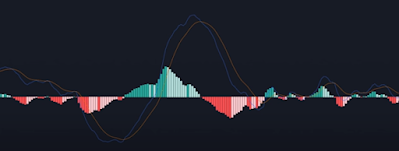# BEST MACD Trading Strategies [90% Win Rate]

The MACD indicator strategy that we will explain is one of the strategies with the highest win rate. You can use this strategy in forex trading and binary options. This method is straightforward to use for you for novice traders. In addition, this method can work in almost every market, and most importantly it can make money by using this MAD indicator strategy.

## MACD indicator

The MACD indicator or can also called (Moving Average Convergence Divergence) is one of the best and most popular technical indicators. This indicator is often used by experienced traders or beginners. This MACD indicator uses moving averages to find market trends, which is also very good.

### How MACD indicator works for profitMACD indicator

The first thing you have to do is add those MACD indicators. After the MACD indicator is added, ensure you know how the MACD indicator actually works so that you can get an advantage in trading. You need to know that the MACD indicator consists of 4 different components, among which are:
1. MACD line
2. Signal line
3. Histogram Line
4. Zero line
• In many cases, usually, the MACD line is a moving average of 12 days.
• The signal line, which is the orange line, is an average of 26 days.
• Then next is the histogram line representing the difference between the MACD line and the signal. So for example, the smaller the space between two lines, the smaller the histogram will be. And vice versa. In addition, We can also see when the MACD line crosses above the signal line, the histogram turns green and if the MACD line crosses below the signal gais it turns red.
• Zero line, this line basically represents the center of the MACD indicator.
"Until now that you know from the 4 components in the MACD indicator, let's make sure to know how to make it work."

The MACD indicator is excellent at finding trends in the market. The way to know if the chart will have an uptrend is the way We look for an upward cross between the MACD line and the signal line. For example, here the MACD cuts above the signal line indicating the chart are in upward momentum, and here the MACD cuts below the signal line indicating that the chart is in downward momentum.  In addition, you can also use a histogram to show how much momentum it really is. So, if the histogram is getting bigger and bigger, it means that there is an increase in momentum. And vice versa.

So, how you want to use this indicator is when the lines intersect upwards, but only if the lines intersect below the zero line. If they cross and are above the zero line, here you will not enter the trade. This is the same as shorting, you only enter a short trade if the line is a downward intersection, and above the zero line.

As we have said before, this indicator is easy to use. But you need to know that the problem that most traders face is that they use this indicator by itself. Actually, the MACD indicator works very well if the market is in a trend. For example here, the MACD indicator works well to show when the price will move up. Because the chart is in an uptrend. But here the price is in a downward trend, but the MACD still gives a signal to buy long even though the price is falling.

To fix this problem, for example, if you are a long trader, you only want to trade if the market is in an uptrend only. You never want to trade against the trend, because the odds will be against you. So, an easy way to find out if the market is in an uptrend. You only need to add a moving average of 200 days. After adding it, you will only see one line as shown below.

If the price is above that line, it means that the market is in an uptrend. If the price is above or below that line, and vice versa, for example, the figure below is an uptrend and a downtrend.

Jadi setelah mengetahui bahwa Kita hanya ingin membeli saat pasar sedang uptrend, jika Kita sedang long tentunya. Jika Kita menggabungkan semua ini, kita kembali jika garis MACD melintas di bawah garis nol, dan harga saat ini juga berada di atas rata-rata pergerkan 200.

This will guarantee that you only trade when the market is in an uptrend, which is a very strong combo. If you just want to shorten, you will only do the opposite. make sure the price is at an average of 200 and the MACD line intersects downwards above the zero line.